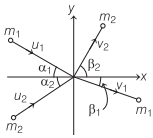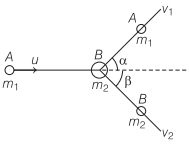# Collisions in Two Dimensions | Definition, Formulas – Work, Energy and Power

Two Dimensional Collision Physics or Oblique Collision Definition:
If the initial and final velocities of colliding bodies do not lie along the same line, then the collision is called two dimensional or oblique collision.

We are giving a detailed and clear sheet on all Physics Notes that are very useful to understand the Basic Physics Concepts.

## Collisions in Two Dimensions | Definition, Formulas – Work, Energy and Power

In horizontal direction,
m1u1 cos α1 + m2u2 cos α2 = m1v1 cos β1 + m2v2 cos β2In vertical direction,
m1u1 sin α1 – m2u2 sin α2 = m1v1 sin β1 – m2v2 sin β2
If m1 = m2 and
α1 + α2 = 90°
then
β1 + β2 = 90°

If a particle A of mass m1 is moving along X-axis with a speed u and makes an elastic collision with another stationary body B of mass m2, thenFrom conservation law of momentum,
m1u = m1v1 cos α + m2v2 cos β
0 = m1v1 sin α – m2v2 sin β

Work, Energy and Power:
Work, energy and power are the three quantities which are inter-related to each other. The rate of doing work is called power. An equal amount of energy is consumed to do a work. So, basically the power is the rate at which energy is consumed to complete a work.